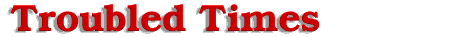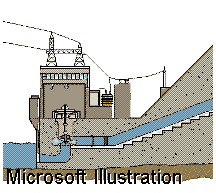#Compare CostsGasoline generators can provide power. Each generator cost about \$500 and has a useful life of about two years. It consumes an average of 1.5 gallons of gasoline each day. The annual operating cost is estimated to be \$852. (Assuming gasoline is \$1.10 per gallon @ 1.5 gallons per day, that comes to \$602 dollars per year. Depreciation is \$250 per year.)

A solar electric system providing equivalent power would require an array of 40, 47 watt panels that would operate an average of five hours per day in the average area. This system would have cost in excess of \$12,000! The estimated life of solar electric panels is 20 years. If the \$12,000 installation cost is prorated over the twenty year life, then the cost becomes \$600 per year.

The annual operating costs for the micro-hydroelectric system are very low. The standard V pulley belts must be replaced every six months, this is approximately \$70 per year. Presently, the bearings are replaced each year at a cost of around \$60. The brushes and bearings in the alternator are replaced annually for about \$20. (I have estimated the alternator operates for the equivalent of almost 400,000 miles per year.) The pulley system is replaced every two years, the estimated annual cost is \$25. The total estimated annual operating cost is \$175.